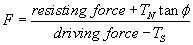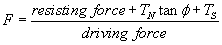The Rocscience International Conference 2021 Proceedings are now available. Read Now

# How Bolts are Implemented in SWedge

Bolts are implemented in the SWedge stability analysis as follows:

## Capacity and Orientation

• Bolts affect the Safety Factor through their Capacity and Orientation (Trend / Plunge). See Bolt Support Force for how bolt capacities are calculated.
• Bolt capacities and orientations are added vectorially, and are included in the Safety Factor calculation as a single, equivalent force passing through the centroid of the wedge. Either the ACTIVE or PASSIVE bolt model will apply (see below).
• Multiple bolts with the same orientation can therefore be simulated by a single bolt having the same total capacity.

## Length and Location

• If the Anchored Length of a bolt = 0 (i.e. it does not pass through the wedge), then the bolt will have NO effect on the model (i.e. its effective capacity will be zero).
• The Location of bolts (on the face of the wedge) has some effect on Safety Factor. Even though all forces in the wedge stability analysis are assumed to pass through the centroid of the wedge, the exact location of a bolt can affect the anchor length of the bolt. Where a selected bolt property type utilizes anchor length for its bolt capacity calculations, the Factor of Safety can be affected.

## Bolts vs. External Force

• If the Bolt Model is Active, a bolt is exactly equivalent to an External Force with the same magnitude and orientation.

TIP: As a suggested exercise you can verify that a bolt using the ACTIVE bolt model and an equivalent External Force, result in the same Safety Factor for a given wedge.

• If the Bolt Model is Passive, a bolt, and an External Force with the same magnitude and orientation, will NOT be equivalent.

## Multiple Bolts

Installation of multiple bolts is useful for visualizing the practical problems of bolt installation, and the necessary bolt lengths and spacing. Or for back-calculating the Safety Factor of an existing wedge support system.

However, since bolts in SWedge simply behave as force vectors passing through the centroid of the wedge, the effect of multiple bolts on the Safety Factor can be simulated by:

• a fewer number of bolts, or even a single bolt, with equivalent capacity and direction, or
• an equivalent External Force
• a support pressure with the Pressure option (this is the recommended way to model pattern bolting in SWedge)

## Active and Passive Bolt Models

In general terms, the Factor of Safety is defined as the ratio of the forces resisting motion, to the driving forces. Driving forces include the mass of the wedge accelerated through gravity, seismic forces, and water pressure. Resisting forces arise from the cohesion and frictional strength of the wedge sliding planes.

Active Support is included in the SWedge analysis as shown in Eqn. 1.Eqn.1

Active Support is assumed to act in such a manner as to DECREASE the DRIVING FORCE in the Factor of Safety calculation. Tensioned cables or rock bolts, which exert a force on the wedge before any movement has taken place, could be considered as Active support.

NOTE: External Forces are incorporated in the SWedge analysis in the same manner as Active Support (i.e. according to Eqn. 1).

Passive Support is included in the SWedge analysis as shown in Eqn. 2.Eqn.2

By this definition, Passive Support is assumed to INCREASE the RESISTING FORCE provided by shear restraint, in the Factor of Safety equation.

Untensioned dowels or grouted cable bolts, which only develop a resisting force after some movement of the wedge has taken place, could be considered as Passive support.

Since the exact sequence of loading and movement in a rock slope is never known in advance, the choice of Active or Passive bolt models is somewhat arbitrary. The user may decide which of the two models is more appropriate for the wedge being analyzed. In general, Passive support will always give a lower Factor of Safety than Active support.